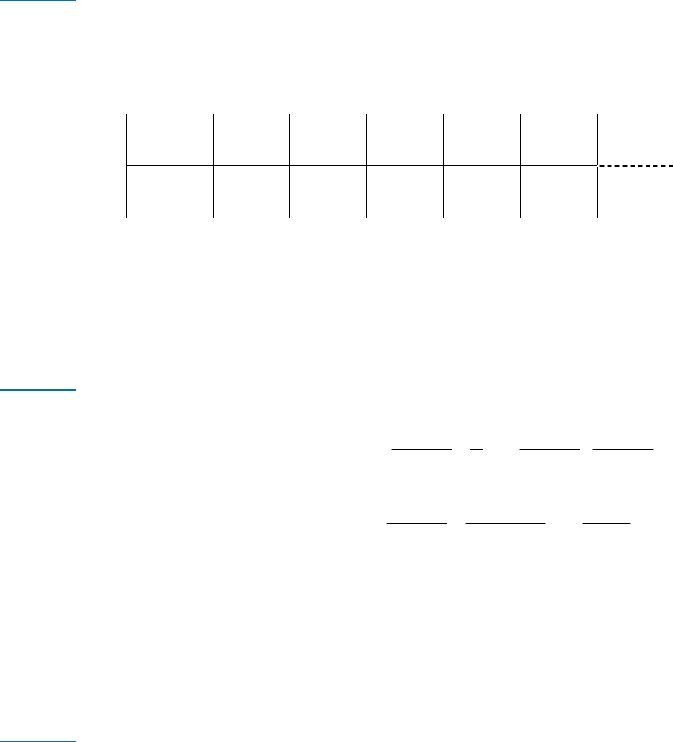Study Guides (380,000)
CA (150,000)
UTSG (10,000)
Midterm

# RSM333H1 Study Guide - Midterm Guide: Cash Conversion Cycle, Dividend, Dividend Policy

Department
Rotman Commerce
Course Code
RSM333H1
Professor
Sabrina Buti
Study Guide
Midterm

This preview shows pages 1-3. to view the full 9 pages of the document.RSM333 – FINAL Exam - Spring 2015
SOLUTIONS
M333H1S Final Exam – Spring 2015 Page 1 of 9
QUESTION 1: COST OF CAPITAL (20 Marks)
1. A firm has 26,000 shares outstanding that are currently trading at \$25, the book value of equity is
\$700,000. Moreover, the company has perpetual bonds with a book value of \$350,000 and a coupon
rate of 5%. The current dividend level of \$3 per share is expected to grow at an annual rate of 4%.
The current yield on government T-bills is 2%, the firm can issue perpetual bonds at a 3% premium
over the current risk-free rate and its tax rate is 40%.
The firm is considering a project to be funded out of internally generated funds that will not alter the
firm’s overall risk. This project requires an initial investment of \$12 million and promises to generate
net annual after-tax cash flows of \$2 million perpetually.
Should this project be undertaken? (10 Marks)
Solution
The market value of equity is =\$25×26,000=\$650,000, the market value of debt is equal to the
book value, =\$350,000, since the coupon payment on bonds (5%) is the same as the current market
rate,=+3%= 2%+3%=5%. Therefore, the company value is =\$650,000+\$350,000=
\$1,000,000, and
=65% and
=35%.
Determine the firm’s cost of equity using the constant dividend growth model:
=
+=\$3(1.04)
\$25 +0.04=16.48%
Solve for the firm’s weighted average cost of capital:
 =
+
(1−)=0.65×16.48%+0.35×5%×(1−0.4)=11.76%
Compute the project’s NPV:  = −12,000,000+\$,,
. =\$5,006,803
The NPV is positive and the project should be undertaken.
2. Firm ABC’s capital structure is \$20 million debt and \$80 million common equity (both are market
values). The cost of equity is 15%, and the cost of debt is 6.1%, so its weighted average cost of capital
is 13.22%. There are no taxes and no bankruptcy costs.
Firm ABC is considering a new project Z to expand its activities whose risk is similar to the risk of the
business currently operated by ABC. Project Z will cost \$2 million and will be entirely financed by
issuing new debt at a 6.1% interest rate. Floatation costs are 0.02% if the debt is underwritten by
EasyCredit, the historical bank of ABC, and 0% if ABC chooses to work with the new investment bank
JP Brothers.
a. Find the cost of new debt if ABC chooses EasyCredit as underwriter and if ABC chooses JP
Brothers as underwriter. (3 Marks)
Solution
If the underwriter is JP Brothers, there are no floatation costs and =6.1%.

Only pages 1-3 are available for preview. Some parts have been intentionally blurred.RSM333H1S Final Exam – Spring 2015 Page 2 of 9
If Easycredit is the underwriter, the cost of debt should be adjusted to include the 2% floatation costs:
=.%
.% =6.22%.
b. Find the weighted average cost of capital of ABC after new debt is issued assuming that ABC
wants to minimize floatation costs. (3 Marks)
Solution
The WACC after issuing the new tranche of debt remains the same (no taxes, no bankruptcy costs and no
floatation costs), i.e., 13.22%.
c. What is the discount rate that firm ABC should use to calculate the NPV of project Z assuming
that ABC chooses JP Brothers as underwriter? Explain. (4 Marks)
Solution
The discount rate should be 13.22% and NOT the cost of debt 6.1%. Even if the project is fully financed by
debt, the shareholders of ABC implicitly guarantee this debt. A discount rate of 6.1% does not take into
account the additional compensation expected by the shareholders who assume the risk of project Z given
that debtholders do not participate in the risk of the company.

Only pages 1-3 are available for preview. Some parts have been intentionally blurred.RSM333H1S Final Exam – Spring 2015 Page 3 of 9
QUESTION 2: PROJECT EVALUATION/WORKING CAPITAL MANAGEMENT (20 Marks)
1. You are considering making a movie. The movie is expected to cost \$10 million up front and take a
year to make. After that, it is expected to make \$5 million in the year it is released and \$2 million for
the following four years. Assume that cash flows are distributed at the end of the year.
a. What is the simple payback period of this investment? If you require a payback period of two
years, will you make the movie? (4 Marks)
Solution
Timeline:
0 1 2 3 4 5 6
–10 0 5 2 2 2 2
It will take 5 years to pay back the initial investment, so the simple payback period is 5 years. You will not
make the movie.
b. What is the movie's NPV if the cost of capital is 10%? Will you make the movie? (3 marks)
Solution
() ()()
() ()
242
224
52 1 1
NPV 10 1
111
52 1
10 1
0.01(1.1)
11.1
\$628,322
r
rrr
r


=− + +

+++



=− + +

+
=−
Also using the NPV methodology, we will not make the movie.
c. Given the NPV that you have calculated, estimate the range you think IRR will be at? (3 Marks)
Solution
When evaluating an independent project, NPV and IRR always result in the same accept/reject decision.
Since the NPV is negative when the cost of capital is 10%, then the IRR of the project must be lower than
10% (otherwise we will accept the project and the decision would not be consistent with the rejection
decision based on the NPV methodology).
###### You're Reading a Preview

Unlock to view full version